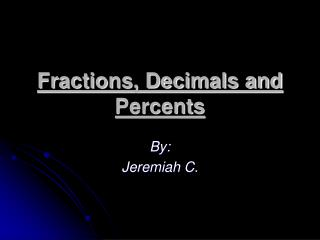DownloadDownload PresentationFractions, Decimals and Percents

# Fractions, Decimals and Percents

Télécharger la présentation## Fractions, Decimals and Percents

- - - - - - - - - - - - - - - - - - - - - - - - - - - E N D - - - - - - - - - - - - - - - - - - - - - - - - - - -
##### Presentation Transcript

1. Fractions, Decimals and Percents By: Jeremiah C.

2. Fractions, Decimals and Percents To decimal First, you divide the bottom to the top Ex: N/D Converting a Fraction 1/5 Answers Decimal- .50 Fraction- 50% To percentage Second, you move the decimals 2 (two) places to the right

3. Fractions, Decimals and Percents Converting a Percent 50% Answers Decimal- .50 Fraction- 1/5 To a decimal First , you move the decimal 2 (two) places to left To a fraction Second, you bring down the divisor (/) and add two (2) zeros then you simplify (cutting down)

4. Fractions, Decimals and Percents Converting a Decimal .50 Answers Percent -50% Fraction -1/5 To percentage First, move decimal two (2) places to the right To a fraction Second, you bring down the divisor(/) and add two zeros, then simplify